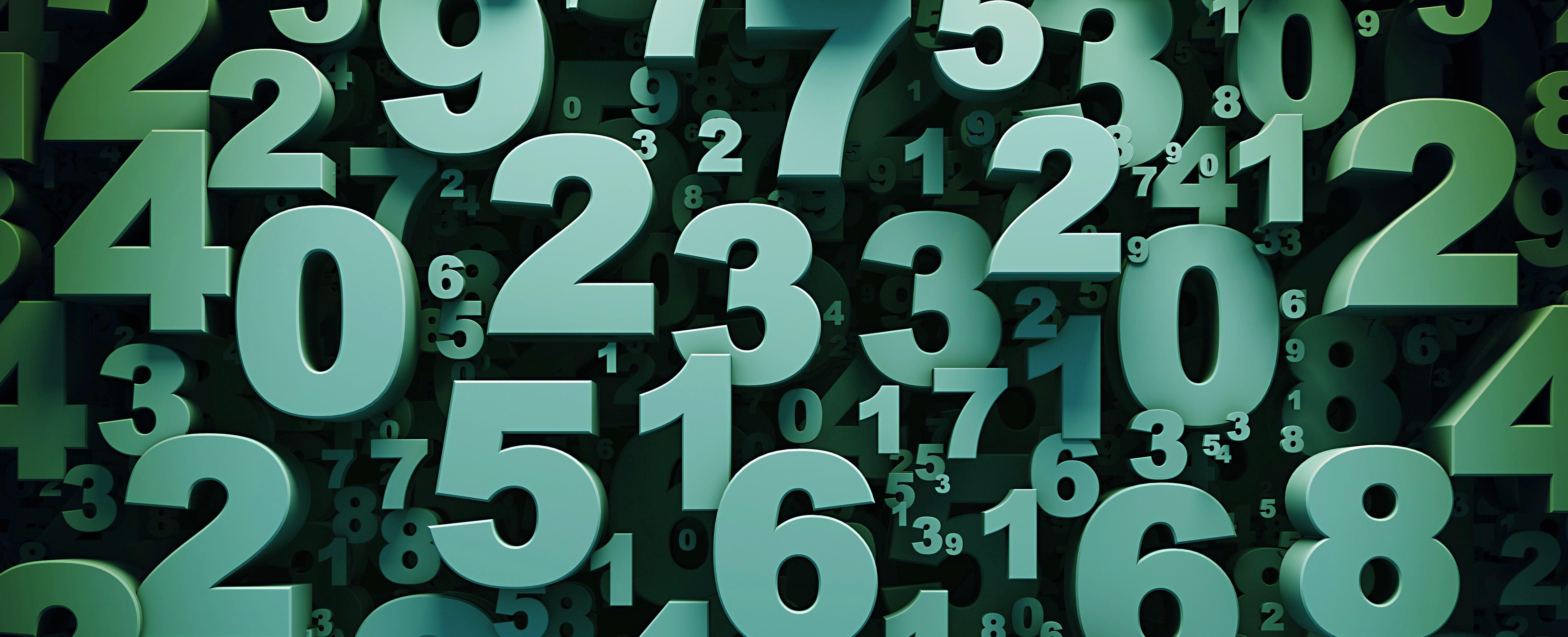# Are there more fractions or decimals?Thanks to Anonymous 9th Grade Student for the question. This is actually a surprisingly deep question. When students are in elementary school, they are taught how to convert between the decimal and fractional representations of a number. 0.4 = 2/5. 0.33333333333…=1/3. 0.237 = 237/1000. So we might initially think that every decimal can be written as a quotient of integers and vise versa. (Integers are whole numbers and negative whole numbers: 4, -3, 25745, -342 and NOT 3.2) This would lead us to believe that there are as many fractions as decimals. We might also recognize that there are an infinite number of fractions and an infinite number of decimals. From there might assume there are the same number of each: infinity.

When students enter middle school, they first encounter irrational numbers. Unlike rational numbers, irrational numbers are, by definition, numbers which cannot be written as a fraction with an integer numerator and denominator. Examples of irrational numbers are π, the square root of 2, and the mathematical constant e. You could write π as fraction π/1 but there is no way to represent it without having a decimal in the numerator or denominator.

All real numbers can be written in decimal form, but some real numbers(irrationals) cannot be written as the fraction of two integers. So, the answer to this question depends on how you define “fraction.” If you define a “fraction” as any number divided by any number, then you could write any irrational number as a fraction by dividing it by one (π/1) and you would have the same number of fractions as decimals. If we define a “fraction” as an integer divided by an integer, there are more decimals than fractions because irrational numbers cannot be written as fractions.

Let’s assume we mean fractions to mean rational numbers. Now let’s go back to the argument that there are an infinite numbers of fractions and decimals so there are the same number(infinity) in each set. Not all “infinities” are the same size though. Using advanced mathematics, there are ways to determine whether one “infinity” is larger than another. Although there is an infinite number in each set, mathematicians would say there is a countable number of fractions (rational numbers) and an uncountable number of decimals(real numbers). Thus, there are more real numbers(decimals) than rational numbers(fractions).

What do “countable” and “uncountable” mean? Suppose we want to know if there are more boys than girls in a room. We could count the boys and girls separately and compare the numbers. We could also have every girl stand next to boy in a one to one correspondence and see if we have extra boys. For comparing mathematical sets with an infinite number of elements, we can follow a similar procedure. If a function exists that could pair every element in a given infinite set in a one to one correspondence with the counting numbers (1,2,3, etc.) then that given set is also countable. (All boys and girls in the room are matched up.) If a one to one correspondence with the counting numbers cannot not be found, then the given set is uncountable. (There are extra boys, so the sets are not the same size.) For a proof that the real numbers are uncountable, look up “Cantor’s Diagonal Argument”.

Another way to look at this problem is to consider long division. When you divide one whole number by another using the long division algorithm the number of possible remainders is the number of whole numbers less than the denominator. When you divide 323 by 7, the only possible remainders after each step are 6, 5, 4, 3, 2, 1 or 0. So you will either eventually get a remainder of zero and the decimal expansion ends or you get the same remainder twice and the decimal expansion starts repeating. (An example of a repeating decimal would be 0.123456565656565656565656565656… where the “56”s will go on forever.) So all quotients of whole numbers (or integers) eventually terminate or repeat. I could however make up a decimal that does not terminate or repeat.For example, 0.010110111011110111110….. This decimal has 1 one, zero, 2 ones, zero, 3 ones, zero, 4 ones, zero, etc. This decimal does not terminate or start repeating itself so it cannot be a rational. Therefore there are more decimals than rational numbers because 0.010110111… is a decimal and not a rational.